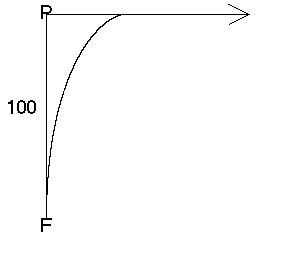All about flooble | fun stuff | Get a free chatterbox | Free JavaScript | Avatarsperplexus dot infoThe farmer and the pig (Posted on 2008-11-13)A farmer wants to catch a pig which is 100 meters away from him. They start running at the same time. The pig runs straight ahead, initially perpendicular to the line farmer-pig, at constant speed. The farmer always runs in the direction where he sees the pig (also at constant speed).If the ratio of the speeds is 3:1 (farmer:pig), how far would the pig have traveled till the farmer caught it?

 See The Solution Submitted by pcbouhid No RatingComments: ( Back to comment list | You must be logged in to post comments.)Steps to solve- to lazy to complete| Comment 2 of 5 |This requires differential equations:

firstly, assign the pig the position Pig( P*t , 0)

where P is the speed of the pig in meters. for some time t.

then assign the position of the farmer at any time Farmer(x,y)

since the farmers path is always directed at the pig

then the equation for the farmer is dy/dx = (-y)/(p*t-x)

Also we know that the speed of the farmer F is

F= sqrt((dx/dt)^2 + (dy/dt)^2)

With some manipulations you will get an equation that has P/F then you just substitute the ratio in and solve.

A differential equation trick that you might want to employ is let J=dy/dx. It is one way you might go about solving the D.E

 Posted by alex on 2008-11-14 09:02:57Please log in:

 Search: Search body:
Forums (0)